### ParallelogramThe etymology (in Greek παραλληλ-όγραμμον, a shape `of parallel lines`) reflects the definition. ==Special cases== ==Characterizations== A simple (non self-intersecting) quadrilateral is a parallelogram if and only if any one of the following statements is true: ==Properties== ==Area formula== ==Proof that diagonals bisect each o.....
Found on http://en.wikipedia.org/wiki/Parallelogram

### Parallelogram• (n.) A right-lined quadrilateral figure, whose opposite sides are parallel, and consequently equal; -- sometimes restricted in popular usage to a rectangle, or quadrilateral figure which is longer than it is broad, and with right angles.
Found on http://thinkexist.com/dictionary/meaning/parallelogram/

### parallelogram<geometry> A right-lined quadrilateral figure, whose opposite sides are parallel, and consequently equal; sometimes restricted in popular usage to a rectangle, or quadrilateral figure which is longer than it is broad, and with right angles. Parallelogram of velocities, forces, accelerations, momenta, etc. ... <mechanics> A parallelogram...
Found on http://www.encyclo.co.uk/local/20973

### parallelogram(from the article `vector`) ...or subtracted. For example, to add or subtract vectors v and w graphically ( the diagram), move each to the origin and complete the parallelogram ...
Found on http://www.britannica.com/eb/a-z/p/17

### parallelogramnoun a quadrilateral whose opposite sides are both parallel and equal in length
Found on https://www.encyclo.co.uk/local/20974

### ParallelogramPar`al·lel'o·gram noun [ Greek ...; ... parallel + ... to write: confer French parallélogramme . See Parallel , and -gram .] (Geom.) A right-lined quadrilateral figure, whose opposite sides are parallel, and consequently equal; -- sometimes restricted in popular usage to a...
Found on http://www.encyclo.co.uk/webster/P/19

### Parallelogram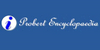A parallelogram is a quadrilateral (four-sided) figure, whose opposite figures are parallel, and consequently equal, but whose angles are not right angles.
Found on http://www.probertencyclopaedia.com/browse/GP.HTM

### parallelogram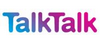A quadrilateral (a shape with four sides) with opposite pairs of sides equal in length and parallel, and opposite angles equal. The diagonals of a parallelogram bisect each other. Its area is the product of the length (l) of one side and the perpendicular distance (height ...
Found on http://www.talktalk.co.uk/reference/encyclopaedia/hutchinson/m0006711.html

### parallelogramA quadrilateral (four-sided figure) whose opposite sides are parallel, and whose opposite angles, therefore, are equal. The diagonals of a parallelogram bisect each other. A parallelogram of base b and height h has an area: A = bh = ab sinA = ab sinB. The height of a parallelogram is h = a sinA...
Found on http://www.daviddarling.info/encyclopedia/P/parallelogram.html

### ParallelogramA quadrilateral in which opposite sides are parallel.
Found on http://www.diracdelta.co.uk/science/source/p/a/parallelogram/source.html

### parallelogram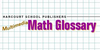A quadrilateral whose opposite sides are parallel and congruent
Examples: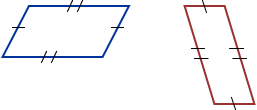Found on http://www.hbschool.com/glossary/math2/index6.html

### parallelogram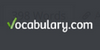a quadrilateral whose opposite sides are parallel and equal
Found on https://www.vocabulary.com/lists/311755

### parallelogram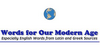parallelogram A four-sided plane figure in which both pairs of opposite sides are parallel and of equal length, and the opposite angles are equal.
Found on http://www.wordinfo.info/words/index/info/view_unit/4033/4

### parallelogram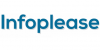parallelogram, closed plane figure bounded by four line segments, or sides, with opposite pairs of sides parallel and equal in length. The rhombus, rectangle, and square are special types of parallelograms. Any side of a parallelogram is a base; an altitude is the perpendicular distance from a base ...[n] - a quadrilateral whose opposite sides are both parallel and equal in length